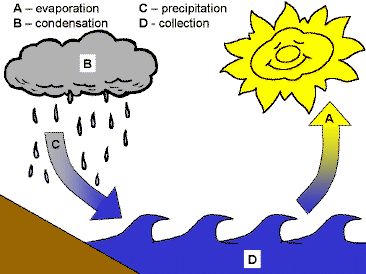# SOF - NSO PDF Sample Papers for Class 9

Resources:

Class 9 sample paper & practice questions for National Science Olympiad (NSO) level 1 are given below. Syllabus for level 1 is also mentioned for these exams. You can refer these sample paper & quiz for preparing for the exam.Q.1 Q.2 Q.3 Q.4 Q.5 Q.6 Q.7 Q.8 Q.9 Q.10
 Q.1 Which of these is the largest living invertebrate phylum? a) Arthropoda b) Annelida c) Mollusca d) Echinodermata
 Q.2 Arrange the given words in ascending order. 1. Dictionary   2. Alphabets   3. Synonyms    4. Encyclopedia    5. Library a) 1, 3, 2, 5, 4 b) 2 ,3, 1, 5, 4 c) 2, 3, 1, 4, 5 d) 2, 1, 3, 4, 5
 Q.3 If the resultant of applied forces is equal to zero, then what force does it resemble? a) Unbalanced force b) Balanced force c) Both a and b d) None of the above
 Q.4 Law of constant proportion is also known as _______________. a) Law of definite proportions b) Proust’s Law c) Both a and b d) None of the above
 Q.5 If R stands for addition, S stands for subtraction, T stands for multiplication, U stands for division, V stands for equal to, W stands for greater than, and X stands for less than. Choose the correct answer: a) 15 U 5 R 3 V 2 T 3 b) 15 S 5 T 3 W 2 R 3 c) 15 U 5 W 3 R 2 T 3 d) 15 R 5 U 3 V 2 R 3
 Q.6 Fill in the blank: The atom becomes ____________, when it gains an electron. a) negatively charged b) positively charged c) remains neutral d) Can't say
 Q.7 Find the number of moles of sodium nitrate which contains 1.5 moles of oxygen atoms. a) 0.5 moles b) 1.5 moles c) 2.0 moles d) 1.0 moles
 Q.8 What is the purpose of cattle farming? a) Milk production b) Farm labour c) Both a and b d) None of the above
 Q.9 Which type of cycle does this image represent?a) Biogeochemical cycle b) Nitrogen cycle c) Carbon cycle d) Water cycle
 Q.10 The rectangular components of a force are applied to move a body. One of the components is 30 N. What should be the other component to obtain a resultant force of 50 N? a) 30 N b) 40 N c) 50 N d) 90 NSample PDF of SOF - National Science Olympiad (NSO) PDF Sample Papers for Class 9: